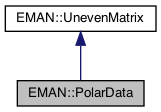EMAN2
EMAN::PolarData Class Reference

a specialized image class for storing the results of a transform from EMData to polar coordinates, currently support 2D only. More...

`#include <polardata.h>`

Inheritance diagram for EMAN::PolarData:[legend]
Collaboration diagram for EMAN::PolarData:[legend]

## Public Member Functions

PolarData ()

PolarData (EMData *image, int xcen, int ycen, string mode)
Construct a PolarData object from a EMData. More...

virtual ~PolarData ()Public Member Functions inherited from EMAN::UnevenMatrix
UnevenMatrix ()

virtual ~UnevenMatrix ()

int get_xsize (int y)
get the x dim size for a given y More...

int get_xmin (int y)
get the minimal x dim value for a given y More...

int get_xmax (int y)
get the maximal x dim value for a given y, note: x1 is one out of the max More...

int get_size ()
get the total size of the data block More...

## Private Member Functions

vector< int > Numrinit (int first_ring, int last_ring, int skip, string mode)
calculate the number of element for each ring More...

int log2 (int n)
Returns the smallet power by which 2 has to be raised to obtain an integer kess equal n. More...

vector< float > ringwe (vector< int > numr, string mode)
calculate ring weights for rotational alignment More...

## Private Attributes

map< int, float > weight
the ring weights for each radius r More...Protected Member Functions inherited from EMAN::UnevenMatrix
void alloc_data ()
allocation memory for data array More...Protected Attributes inherited from EMAN::UnevenMatrix
float * data
store all data in one dimension float array for cache efficiency, we calculate the offset for x, y dimension More...

map< int, Xdimdesc_data
describe for each y, the x dimension's size and range More...

int tot_size
the total size of the data More...

## Detailed Description

a specialized image class for storing the results of a transform from EMData to polar coordinates, currently support 2D only.

data on x dimension may be variable size, which is defined in map< int, Xdim > desc_data

Definition at line 136 of file polardata.h.

## ◆ PolarData() [1/2]

 EMAN::PolarData::PolarData ( )
inline

Definition at line 139 of file polardata.h.

139{printf("Welcome to PolarData class... \n");}

## ◆ PolarData() [2/2]

 PolarData::PolarData ( EMData * image, int xcen, int ycen, string mode )

Construct a PolarData object from a EMData.

Parameters
 image the EMData object to be converted xcen the x dimension of the center ycen the y dimension of the center mode

Definition at line 82 of file polardata.cpp.

83{
84// int nsam = image->get_xsize();
85// int nrow = image->get_ysize();
86
87
88// int nring = numr.size()/3;
89
90}

## ◆ ~PolarData()

 virtual EMAN::PolarData::~PolarData ( )
inlinevirtual

Definition at line 149 of file polardata.h.

149 {
150 printf("Destructor of PolarData...\n");
151 }

## ◆ log2()

 int EMAN::PolarData::log2 ( int n )
private

Returns the smallet power by which 2 has to be raised to obtain an integer kess equal n.

Parameters
 n int
Returns
int

## ◆ Numrinit()

 vector< int > EMAN::PolarData::Numrinit ( int first_ring, int last_ring, int skip, string mode )
private

calculate the number of element for each ring

Parameters
 first_ring the ring number for the first ring last_ring the ring number for the last ring skip step of ring mode half mode('H'/'h') or full mode('F'/'f')
Returns
the vector for number of elements of each ring

## ◆ ringwe()

 vector< float > EMAN::PolarData::ringwe ( vector< int > numr, string mode )
private

calculate ring weights for rotational alignment

Parameters
 numr number of element for each ring mode half mode('H'/'h') or full mode('F'/'f')
Returns
vector<float> the weight for each ring

## ◆ weight

 map< int, float > EMAN::PolarData::weight
private

the ring weights for each radius r

Definition at line 167 of file polardata.h.

The documentation for this class was generated from the following files: